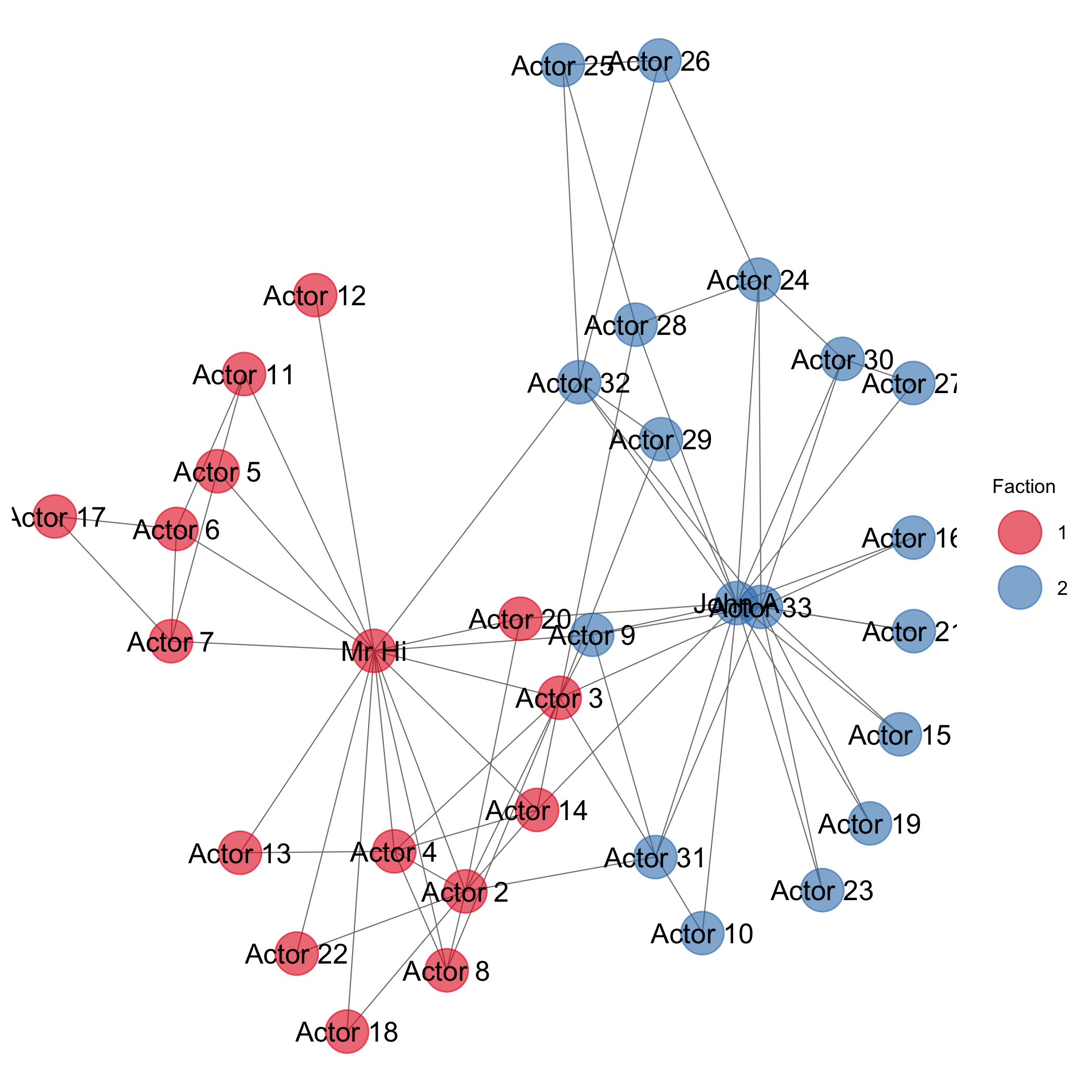# Graph Laplacian Eigenmaps

Graph laplacian eigenmaps (GLE) are one means, among many, of embedding graphs into a low-dimensional numeric space. Specifically, GLE is an approach based on Matrix Factorization that takes as input non-relational data and outputs node embeddings. The key insight of GLEs is that the graph property to be preserved can be interpreted as pairwise node similarities. Thus, a larger penalty is imposed if two nodes with large similarity are embedded far apart. Thus, the goal of this approach is to find an embedding that minimizes this penalty.

The gist of this approach is that it aims to first represent the graph as a Graph Laplacian Matrix, $L$. $L$ is a means of representing a graph in matrix form following the definition $L = D - W$, where $D$ is the degree matrix, a diagonal matrix containing the degree of each node, and $W$ is the adjacency matrix of weights. Under this representation, all positive values correspond to degrees of the node, and all negative values to the weights of the edges. We then perform a decomposition on $L$ to find its eigenvalues. The eigenvectors corresponding to the smallest of the eigenvalues are used as the embedding.

The optimal embedding, $y^{\ast}$ can be derived from the following objective function,

$y^{\ast} = \text{argmin}\_{y} \sum\_{i\neq j} (y\_{i} - y\_{j}^{2} W\_{ij}) = \text{argmin}\_{y} y^{T}Ly$

Where $W_{ij}$ is the similarity matrix between every pair of nodes $v_{i}$ and $v_{j}$. $L$ is the graph laplacian matrix, $D$ is the diagonal matrix for which $D_{ii} = \sum_{i \neq j}W_{ij}$.

The goal of this objective function is to find the embedding, $y^{\ast}$ that minimizes the error compared to the Graph Laplacian Matrix, $L$. One benefit of representing a graph with $L$ rather than a simple adjacency matrix is that the diagonal will contain the degrees of the graph; the larger the degree of a node, the more that its row will be “weighted” when computing the objective function. The practical effect is that larger degree nodes will have a greater impact on the embedding than lower-degree nodes.

There are several expansions on this basic optimization, but in each of these variants, the optimal embedding $y^{\prime}$ are the eigenvectors of the laplacian matrix, $\lambda$, which can be calculated by solving the the eigenproblem $Wy = \lambda Dy$.

Arrange the eigenvalues from smallest to largest. The first eigenvalue should be close to zero, and its corresponding eigenvector is not typically used for embedding. Rather, use the remaining eigenvalues and their corresponding eigenvectors to construct the $d$-dimensional embedding. For example, a 3-dimensional embedding can be constructed using the eigenvectors corresponding to the 2nd, 3rd, and 4th smallest eigenvalues. A 2-dimensional embedding can be constructed using the eigenvectors of the 2nd and 3rd smallest eigenvalues.

Lets walk through this process in R.

Consider the Karate network, shown below, which is a popular network exemplifying community structure.Having loaded this data into R, we can construct the Graph Laplacian Matrix, $L$, from the adjacency matrix $W$ and the degree matrix, $D$.

W <- as.matrix(as.matrix.network(karate))
D <- diag(rowSums(W))
L <- D - W


Then, we can solve the eigenproblem $Wy = \lambda Dy$ and select the eigenvectors corresponding to the 2nd and 3rd smallest eigenvectors to produce an embedding.

eig <- eigen(L, D)
col_dim <- dim(eig$vectors) vectors <- eig$vectors[,(col_dim - 1):(col_dim - 2)]


The resulting embedding is shown below.We can see that much of the structure from the original graph also appears in the embedding. As with the network, the embedding demonstrates a clear division between the two communities. Additionally, we see all the nodes that are directly connected to the opposing community are clustered together. Meanwhile, nodes for Actor 5, 6, 7, 11, and 17 are clustered apart form the other clusters, reflecting their somewhat isolated position in the original graph. Similarly, Actor 12 only maintains one connection to Mr. H, and is thus set far apart from the other nodes in the embedding.

There are many uses for graph embeddings. They allow for fast computation of node distances and can serve as effective features for graph-based classification problems.

This content was largely drawn from the following resources,

Cai, H., Zheng, V. W., & Chang, K. C.-C. (2017). A Comprehensive Survey of Graph Embedding: Problems, Techniques and Applications. ArXiv:1709.07604 [Cs]. Retrieved from https://arxiv.org/abs/1709.07604

Belkin, M., & Niyogi, P. (2001). Laplacian Eigenmaps and Spectral Techniques for Embedding and Clustering. Proceedings of the 14th International Conference on Neural Information Processing Systems: Natural and Synthetic, 585–591. Retrieved from https://dl.acm.org/citation.cfm?id=2980539.2980616

Tags: